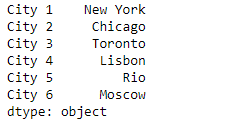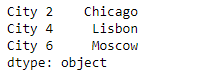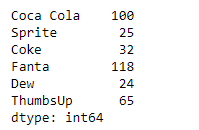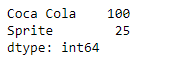# Python | Pandas Series.select()

• Last Updated : 07 Feb, 2019

Pandas series is a One-dimensional ndarray with axis labels. The labels need not be unique but must be a hashable type. The object supports both integer- and label-based indexing and provides a host of methods for performing operations involving the index.

Pandas` Series.select()` function return data corresponding to axis labels matching criteria. We pass the name of the function as an argument to this function which is applied on all the index labels. The index labels satisfying the criteria are selected.

Attention geek! Strengthen your foundations with the Python Programming Foundation Course and learn the basics.

To begin with, your interview preparations Enhance your Data Structures concepts with the Python DS Course. And to begin with your Machine Learning Journey, join the Machine Learning - Basic Level Course

Syntax: Series.select(crit, axis=0)

Parameter :
crit : called on each index (label). Should return True or False
axis : int value

Returns : selection : same type as caller

Example #1: Use `Series.select()` function to select the names of all those cities from the given Series object for which it’s index labels has even ending.

 `# importing pandas as pd``import` `pandas as pd`` ` `# Creating the Series``sr ``=` `pd.Series([``'New York'``, ``'Chicago'``, ``'Toronto'``, ``'Lisbon'``, ``'Rio'``, ``'Moscow'``])`` ` `# Create the Datetime Index``index_ ``=` `[``'City 1'``, ``'City 2'``, ``'City 3'``, ``'City 4'``, ``'City 5'``, ``'City 6'``]`` ` `# set the index``sr.index ``=` `index_`` ` `# Print the series``print``(sr)`

Output :Now we will use `Series.select()` function to select the names of all those cities, whose index label ends with even integer value.

 `# Define a function to  Select those cities whose index``# label's last character is an even integer``def` `city_even(city):``    ``# if last character is even``    ``if` `int``(city[``-``1``]) ``%` `2` `=``=` `0``:``        ``return` `True``    ``else``:``        ``return` `False`` ` `# Call the function and select the values``selected_cities ``=` `sr.select(city_even, axis ``=` `0``)`` ` `# Print the returned Series object``print``(selected_cities)`

Output :As we can see in the output, the `Series.select()` function has successfully returned all those cities which satisfies the given criteria.

Example #2: Use `Series.select()` function to select the sales of the ‘Coca Cola’ and ‘Sprite’ from the given Series object.

 `# importing pandas as pd``import` `pandas as pd`` ` `# Creating the Series``sr ``=` `pd.Series([``100``, ``25``, ``32``, ``118``, ``24``, ``65``])`` ` `# Create the Index``index_ ``=` `[``'Coca Cola'``, ``'Sprite'``, ``'Coke'``, ``'Fanta'``, ``'Dew'``, ``'ThumbsUp'``]`` ` `# set the index``sr.index ``=` `index_`` ` `# Print the series``print``(sr)`

Output :Now we will use `Series.select()` function to select the sales of the listed beverages from the given Series object.

 `# Function to select the sales of ``# Coca Cola and Sprite``def` `show_sales(x):``    ``if` `x ``=``=` `'Sprite'` `or` `x ``=``=` `'Coca Cola'``:``        ``return` `True``    ``else``:``        ``return` `False`` ` `# Call the function and select the values``selected_cities ``=` `sr.select(show_sales, axis ``=` `0``)`` ` `# Print the returned Series object``print``(selected_cities)`

Output :As we can see in the output, the `Series.select()` function has successfully returned the sales data of the desired beverages from the given Series object.

My Personal Notes arrow_drop_up